Refer to our Texas Go Math Grade 6 Answer Key Pdf to score good marks in the exams. Test yourself by practicing the problems from Texas Go Math Grade 6 Lesson 10.1 Answer Key Exponents.

Identifying Repeated Multiplication

A real-world problem may involve repeatedly multiplying a factor by itself.

A scientist observed the hourly growth of bacteria and recorded his observations in a table.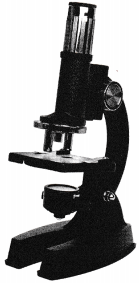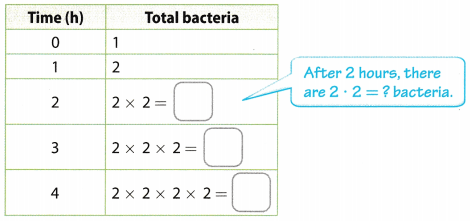(A) Complete the table. What pattern(s) do you see in the Total bacteria column?

(B) Complete each statement.
At 2 hours, the total is equal to the product of two 2s.
At 3 hours, the total is equal to the product of ___________________ 2s.
At 4 hours, the total is equal to the product of ___________________ 2s.

Reflect

Question 1.
Communicate Mathematical Ideas How is the time, in hours, related to the number of times 2 is used as a factor?
The number of times 2 is used as a factor is equal to the time in hours. It can be seen that when t = 1, 2 is used only once, while when t = 2, 2 is used twice and so on.

Use exponents to write each expression.

Question 2.
4 × 4 × 4
4 × 4 × 4

Find the base, or the numbers being multiplied. The base is 4.
Find the exponent by counting the number of 4s being multiplied.
The exponent is 3.

Final Solution:= 43

Question 3.
6 = ___________
6

Find the base, or the numbers being multiplied. The base is 6.
Find the exponent by counting the number of 6s being multiplied.
The exponent is 1

Final Solution: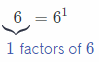Question 4.
$$\frac{1}{8}$$ × $$\frac{1}{8}$$
$$\frac{1}{8}$$ × $$\frac{1}{8}$$

Find the base, or the numbers being multiplied. The base is $$\frac{1}{8}$$.
Find the exponent by counting the number of times $$\frac{1}{8}$$ appears in the expression.
The exponent is 2

Final Solution:Question 5.
5 × 5 × 5 × 5 × 5 × 5
5 × 5 × 5 × 5 × 5 × 5

Find the base, or the numbers being multiplied. The base is 5.
Find the exponent by counting the number of 6s being multiplied.
The exponent is 6.

Final Solution: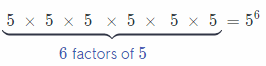Find the value of each power.

Question 6.
34 ____________
Solution to this example is given below = 34
Identify the base and the exponent.
The base s 3, and the exponent is 4

Final Solution:
Evaluate: 34 = 3 × 3 × 3 × 3 = 81

Question 7.
(- 1)9 ___________
Simplify the given expression by identifying the base and exponent
The base is – 1 and the exponent is 9
= (- 1) ∙ (- 1) ∙ (- 1) ∙ (- 1) ∙ (- 1) ∙ (- 1) ∙ (- 1) ∙ (- 1) ∙ (- 1) multiply the case by itself nine times
= – 1
The value of the expression is – 1.

Question 8.
$$\left(\frac{2}{5}\right)^{3}$$ _____________
Solution to this example is given below = $$\left(\frac{2}{5}\right)^{3}$$
Identify the base and the exponent.
The base is $$\frac{2}{5}$$, and the exponent is 3

Final Solution:
Evaluate: $$\left(\frac{2}{5}\right)^{3}=\left(\frac{2}{5}\right) \times\left(\frac{2}{5}\right) \times\left(\frac{2}{5}\right)=\frac{8}{125}$$

Question 9.
– 122 ___________
Simplify the given expression by identifying the base and exponent.
The base is 12 and the exponent is 2.
= – (12 × 12) multiply the base by itself twice
= – 144
The value of the expression is – 144

Question 1.
Complete the table.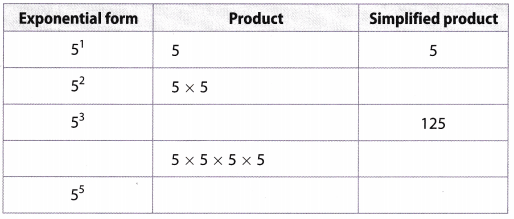Exponential form Product Simplified Product 51 5 5 52 5 × 5 25 53 5 × 5 × 5 125 54 5 × 5 × 5 × 5 625 55 5 × 5 × 5 × 5 × 5 3125

Use an exponent to write each expression.

Question 2.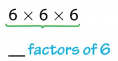6 × 6 × 6
Find the base, or the numbers being multiplied The base is 6
Find the exponent by counting the number of 6s being multiplied.
The exponent is 3.

Final Solution: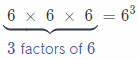Question 3.
10 × 10 × 10 × 10 × 10 × 10 × 10
10 × 10 × 10 × 10 × 10 × 10 × 10
Find the base, or the numbers being multiplied The base is 10
Find the exponent by counting the number of 6s being multiplied.
The exponent is 7.

Final Solution:Question 4.
$$\frac{3}{4} \times \frac{3}{4} \times \frac{3}{4} \times \frac{3}{4} \times \frac{3}{4}$$
Solution to this example is given below
$$\frac{3}{4} \times \frac{3}{4} \times \frac{3}{4} \times \frac{3}{4} \times \frac{3}{4}$$

Find the base, or the numbers being multiplied The base is $$\frac{3}{4}$$
Find the exponent by counting the number of $$\frac{3}{4}$$ appears in.
the expression. The exponent is 5.

Final Solution:Question 5.
$$\frac{7}{9} \times \frac{7}{9} \times \frac{7}{9} \times \frac{7}{9} \times \frac{7}{9} \times \frac{7}{9} \times \frac{7}{9} \times \frac{7}{9}$$
Solution to this example is given below
$$\frac{7}{9} \times \frac{7}{9} \times \frac{7}{9} \times \frac{7}{9} \times \frac{7}{9} \times \frac{7}{9} \times \frac{7}{9} \times \frac{7}{9}$$

Find the base, or the numbers being multiplied The base is $$\frac{7}{9}$$
Find the exponent by counting the number of $$\frac{7}{9}$$ appears in.
the expression. The exponent is 5.

Final Solution:Find the value of each power.

Question 6.
83
Solution to this example is given below 83
Identify the base and the exponent
The base is 8, and the exponent is 3

Final Solution:
Evaluate: 83 = 8 × 8 × 8 = 512

Question 7.
74
Solution to this example is given below 74
Identify the base and the exponent
The base is 7, and the exponent is 4

Final Solution:
Evaluate: 74 = 7 × 7 × 7 × 7 = 2401

Question 8.
103
Solution to this example is given below 103
Identify the base and the exponent
The base is 10, and the exponent is 3

Final Solution:
Evaluate: 103 = 10 × 10 × 10 = 1000

Question 9.
$$\left(\frac{1}{4}\right)^{2}$$
Solution to this example is given below $$\left(\frac{1}{4}\right)^{2}$$
Identify the base and the exponent
The base is $$\frac{1}{4}$$, and the exponent is 4

Final Solution:
Evaluate: $$\left(\frac{1}{4}\right)^{2}=\left(\frac{1}{4}\right) \times\left(\frac{1}{4}\right)=\frac{1}{16}$$

Question 10.
$$\left(\frac{1}{3}\right)^{3}$$
Solution to this example is given below $$\left(\frac{1}{3}\right)^{3}$$
Identify the base and the exponent
The base is $$\frac{1}{3}$$, and the exponent is 3

Final Solution:
Evaluate: $$\left(\frac{1}{3}\right)^{3}=\left(\frac{1}{3}\right) \times\left(\frac{1}{3}\right) \times\left(\frac{1}{3}\right)=\frac{1}{27}$$

Question 11.
$$\left(\frac{6}{7}\right)^{2}$$
Solution to this example is given below $$\left(\frac{6}{7}\right)^{2}$$
Identify the base and the exponent
The base is $$\frac{6}{7}$$, and the exponent is 2

Final Solution:
Evaluate: $$\left(\frac{6}{7}\right)^{2}=\left(\frac{6}{7}\right) \times\left(\frac{6}{7}\right)=\frac{36}{49}$$

Question 12.
0.82
Solution to this example is given below 0.82
Identify the base and the exponent
The base is 0.8, and the exponent is 2

Final Solution:
Evaluate: 0.82 = 0.8 × 0.8 = 0.64

Question 13.
0.53
Solution to this example is given below 0.53
Identify the base and the exponent
The base is 0.5, and the exponent is 3

Final Solution:
Evaluate: 0.53 = 0.5 × 0.5 × 0.5 = 0.125

Question 14.
1.12
Solution to this example is given below 1.12
Identify the base and the exponent
The base is 1.1, and the exponent is 2

Final Solution:
Evaluate: 1.12 =1.1 × 1.1 = 1.21

Question 15
80
Given expression 80
Any power raised to exponent 0 is always equal to 1, therefore:
80 = 1

Question 16.
121
Given expression 121
Any power raised to exponent 1 is equal to the base of the given power, therefore
121 = 12

Question 17.
$$\left(\frac{1}{2}\right)^{0}$$
Given expression $$\left(\frac{1}{2}\right)^{0}$$
Any power raised to exponent 0 is always equal to 1, therefore:
$$\left(\frac{1}{2}\right)^{0}$$ = 1

Question 18.
(- 2)3
Simplify the given expression by identifying the base and exponent
The base is – 2 and the exponent is 3.
= (- 2) × (- 2) × (- 2) multiply the base by itself three times
= – 8
The value of the expression is – 8

Question 19.
$$\left(-\frac{2}{5}\right)^{2}$$
Simplify the given expression by identifying the base and exponent
The base is $$\left(-\frac{2}{5}\right)^{2}$$ and the exponent is 2.
= $$\left(-\frac{2}{5}\right) \times\left(-\frac{2}{5}\right)$$ multiply the base by itself three times
= $$\frac{4}{25}$$
The value of the expression is $$\frac{4}{25}$$

Question 20.
– 92
Simplify the given expression by identifying the base and exponent
The base is 9 and the exponent is 2.
= – (9 × 9) multiply the base by itself three times
= – 81
The value of the expression is – 81

Essential Question Check-In

Question 21.
How do you use an exponent to represent a number such as 16?
The number 16 is written as its factors: 16 = 2 × 2 × 2 × 2 which can be condensed to a power as 24.
The number 16 is written as it other factor: 16 = 4 × 4 which can be condensed to a power as 42,
16 = 24 = 42

Write the missing exponent.

Question 22.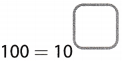Answer:
Solution to this example is given below
100 = 10x
100 = 102 (Convert 100 to base 10)
102 = 10x (Divide both sides by 10)
2 = x
x = 2
100 = 102
The missing exponent is 2

Question 23.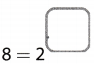Solution to this example is given below
8 = 2x
8 = 23 (Convert 8 to base 2)
23 = 2x (Divide both sides by 2)
3 = x
x = 3
8 = 23
The missing exponent is 3

Question 24.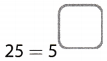Solution to this example is given below
25 = 5x
25 = 52 (Convert 25 to base 5)
52 = 5x (Divide both sides by 5)
2 = x
x = 2
25 = 52
The missing exponent is 2

Question 25.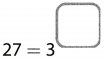Solution to this example is given below
27 = 3x
27 = 33 (Convert 27 to base 3)
33 = 3x (Divide both sides by 3)
3 = x
x = 3
27 = 33
The missing exponent is 3

Question 26.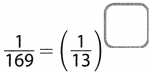Given expression:
$$\left(\frac{1}{169}\right)=\left(\frac{1}{13}\right)^{x}$$
Rewrite the given expression by writing the left hand side of the equation as a power of base $$\left(\frac{1}{13}\right)$$, therefore:
$$\left(\frac{1}{13}\right)^{2}=\left(\frac{1}{13}\right)^{x}$$
Now the bases are same, so compare the exponents to evaluate x, therefore:
x = 2
So the expression becomes:
$$\left(\frac{1}{169}\right)=\left(\frac{1}{13}\right)^{2}$$

Question 27.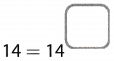Given expression:
14 = 14x
Since the number on both sides of the equation is same, this implies that x = 1
So the expression becomes:
14 = 141

Question 28.Solution to this example is given below
32 = 2x
32 = 25 (Convert 32 to base 2)
25 = 2x (Divide both sides by 2)
5 = x
x = 5
32 = 25
The missing exponent is 5

Question 29.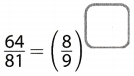Solution to this example is given below
$$\frac{64}{81}=\left(\frac{8}{9}\right)^{x}$$
$$\frac{64}{81}=\left(\frac{8}{9}\right)^{2}$$ (Convert $$\frac{64}{81}$$ to base $$\frac{8}{9}$$)
$$\left(\frac{8}{9}\right)^{2}=\left(\frac{8}{9}\right)^{x}$$ (Divide both sides by $$\frac{8}{9}$$)
2 = x
x = 2
$$\frac{64}{81}=\left(\frac{8}{9}\right)^{2}$$
The missing exponent is 2

Write the missing base.

Question 30.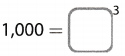Solution to this example is given below
1000 = x3
1000 = 103 (Convert 1000 to base 10)
103 = x3
10 = x
x = 10
1000 = 103
The missing base is 10

Question 31.Solution to this example is given below
256 = x4
256 = 44 (Convert 256 to base 4)
44 = x4
4 = x
x = 4
256 = 44
The missing base is 4

Question 32.Solution to this example is given below
16 = x4
16 = 24 (Convert 16 to base 2)
24 = x4
2 = x
x = 2
16 = 24
The missing base is 2

Question 33.Solution to this example is given below
9 = x2
9 = 32 (Convert 9 to base 3)
32 = x2
3 = x
x = 3
9 = 32
The missing base is 3

Question 34.Solution to this example is given below
$$\frac{1}{9}$$ = x2
$$\frac{1}{9}$$ (Convert $$\frac{1}{9}$$ to base $$\frac{1}{3}$$)
$$\left(\frac{1}{3}\right)^{2}$$ = x2
$$\frac{1}{3}$$ = x
x = $$\left(\frac{1}{3}\right)^{2}$$
$$\frac{1}{9}=\left(\frac{1}{3}\right)^{2}$$
The missing base is $$\frac{1}{3}$$.

Question 35.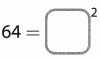Solution to this example is given below
729 = x2
729 = 82 (Convert 729 to base 9)
93 = x2
9 = x
x = 9
729 = 93
The missing base is 9

Question 36.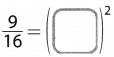Solution to this example is given below
$$\frac{9}{16}$$ = x2
$$\frac{9}{16}=\left(\frac{3}{4}\right)^{2}$$ (Convert $$\frac{9}{16}$$ to base $$\frac{3}{4}$$)
$$\left(\frac{3}{4}\right)^{2}$$ = x2
$$\frac{3}{4}$$ = x
x = $$\left(\frac{3}{4}\right)$$
$$\frac{9}{16}=\left(\frac{3}{4}\right)^{2}$$
The missing base is $$\frac{3}{4}$$.

Question 37.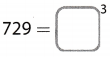Solution to this example is given below
729 = x3
729 = 93 (Convert 729 to base 9)
82 = x2
8 = x
x = 8
64 = 82
The missing base is 8

Question 38.
Hadley’s softball team has a phone tree in case a game is canceled. The coach calls 3 players. Then each of those players calls 3 players, and soon. How many players will be notified during the third round of calls?
1st round = 3 = 31 = 3
2nd round = 3 × 3 = 32 = 9
3rd round = 3 × 3 × 3 = 33 = 27
On the third round of calls, 27 players were notified

Question 39.
Tim is reading a book. On Monday he reads 3 pages. On each day after that, he reads triple the number of pages as the previous day. How many pages does he read on Thursday?
Monday – 3 = 31 = 3
Tuesday – 3 × 3 = 32 = 9
Wednesday – 3 × 3 × 3 = 33 = 27
Thursday – 3 × 3 × 3 × 3 = 34 = 81
Tim was able to read 81 pages on Thursday.

Question 40.
Which power can you write to represent the area of the square shown? Write the power as an expression with a base and an exponent, and then find the area of the square.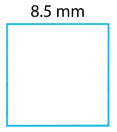Determine the area of the square.
A = s2 formula to get the area of a square
A = (8.5)2 substitute for the given values
Identify the base and the exponent
The base is 8.5 and the exponent is 2.
= 8.5 mm × 8.5 mm multiply the base by itself twice
= 72.25 mm2 area of the square
The area of the square is 72.25 mm2

Question 41.
Antonia is saving for a video game. On the first day, she saves two dollars in her piggy bank. Each day after that, she doubles the number of dollars she saved on the previous day. How many dollars does she save on the sixth day?

• 1st day – 2 = 21 = 82
• 2nd clay – 2 × 2 = 22 = $1 • 3rd day – 2 × 2 × 2 = 23 =$8
• 4th day – 2 × 2 × 2 × 2 = 24 = $16 • 5th day – 2 × 2 × 2 × 2 × 2 = 25 =$32
• 6th day – 2 × 2 × 2 × 2 × 2 × 2 = 26 = $64 Antonia saved$64 on the sixth day.

Question 42.
A certain colony of bacteria triples in length every 10 minutes. Its length is now 1 millimeter. How long will it be in 40 minutes?

• 0 minutes = 1 mm
• 10 minutes = 1 × 3 = 31= 3 mm
• 20 minutes = 3 × 3 = 32 = 9 mm
• 30 minutes = 3 × 3 × 3 = 33 = 27 mm
• 40 minutes = 3 × 3 × 3 × 3 = 34 = 81 mm

After 40 minutes, the length of the bacteria will be 81 mm.

Question 43.
Write a power represented with a positive base and a positive exponent whose value is less than the base.
The base is 4 and the exponent is $$\frac{1}{2}$$.
Evaluate:
$$4^{\frac{1}{2}}$$ = √4 = 2
The expression is $$4^{\frac{1}{2}}$$ = 2.

Question 44.
Which power can you write to represent the volume of the cube shown?
Write the power as an expression with a base and an exponent, and then find the volume of the cube.Determine the volume of the cube.
V = s3 formula to get the volume of a cube
$$\left(\frac{1}{3}\right)^{3}$$ = substitute for the given values

Identify the base and the exponent
The base is $$\frac{1}{3}$$ and the exponent is 3
= $$\frac{1}{3}$$ in × $$\frac{1}{3}$$ in × $$\frac{1}{3}$$ in multiply the base by itself thrice
= $$\frac{1}{27}$$ in volume of the cube
The volume of the cube is $$\frac{1}{27}$$ in3

H.O.T. Focus On Higher Order Thinking

Question 45.
Communicate Mathematical Ideas What is the value of 1 raised to the power of any exponent? What is the value of 0 raised to the power of any nonzero exponent? Explain.
When 1 is raised to any number, the value of that expression is always equal to 1 because of its identity property. (1n = 1)
When 0 is raised to any nonzero exponent, the value of that expression is always zero because of its zero property. (0n = 0 where: n > 0)
The va1ue of 1n = 1 while the value of 0n = 0

Question 46.
Look for a Pattern Find the values of the powers in the following pattern: 101, 102, 103, 104 ……. 106 Describe the pattern, and use it to evaluate 106 without using multiplication.
Given pattern: 101, 102, 103, when expanded according to rules of power expansion reveals the pattern: 10, 100, 1000. 10000…. and according to this 106 = 1000000

Question 47.
Critical Thinking Some numbers can be written as powers of different bases. For example, 81 = 92 and 81 = 34. Write the number 64 using three different bases.
Write the given number as a product of it factor 2, therefore:
64 = 2 × 2 × 2 × 2 × 2 × 2 = 26
Write the given number as a product of it factor 4, therefore:
64 = 4 × 4 × 4 = 43
Write the given number as a product of it factor 8, therefore:
64 = 8 × 8 = 82
64 = 26 = 43 = 82

Question 48.
Justify Reasoning Oman said that it ¡s impossible to raise a number to the power of 2 and get a negative value. Do you agree with Oman? Why or why not?